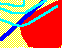7.7 THE CAPM IN EQUILIBRIUM: OVERVIEW

In this section, we re-interpret the CAPM assuming that investors in the capital markets are risk-averse and that markets are in equilibrium.

First, we examine the investment behavior that is consistent with investors who maximize their expected utility. The principal result that emerges from this analysis is the two-fund theorem. This theorem, coupled with market equilibrium, lets us interpret the tangency portfolio, M, as being the value-weighted portfolio of all risky securities. This result supports calling M the market portfolio.

We then examine the implications of risk-aversion on the market portfolio. The two-fund theorem also forms the basis for empirical studies of the CAPM, some of which are discussed at the end of this section.

The results of this section set the stage for deriving the equilibrium value of the firm, where we describe in detail the interaction between supply and demand in the capital market. This analysis will give you insight into how investor tolerances for risk and expected cash flows from firms combine to determine the value of the firm.

previous topic

next topic